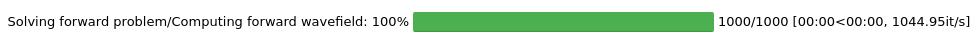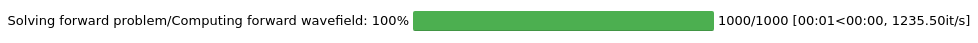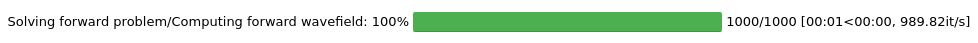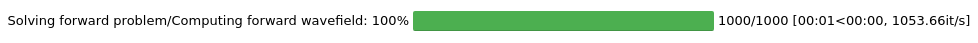Version:

This documentation is not for the latest stable Salvus version.This tutorial is presented as Python code running inside a Jupyter Notebook, the recommended way to use Salvus. To run it yourself you can copy/type each individual cell or directly download the full notebook, including all required files.# Semi-analytic Test - Elastic

• Reference solution: Gar6more2D
• Physics: Elastic wave equation
In this notebook will use Gar6more2D to generate a set of of semi-analytic solutions to the 2-D elastic wave equation, and then compare these solutions to those computed within Salvus. To make things a bit more interesting, we will consider a domain with one stress-free (free-surface) boundary, in an analogue to Lamb's problem in elastic media.
Copy
%config Completer.use_jedi = False
%matplotlib inline

# Stdlib packages
import os
import pathlib
import shutil
import subprocess

# Third party packages
import matplotlib.pyplot as plt
import numpy as np
import obspy
import pyasdf

# Import things from SalvusFlow
from salvus.flow import api

# Configuration helpers from SalvusFlow.
import salvus.flow.simple_config as sc

# And some helper functions to run the integration tests.
from integration_test_mesh_helper import (
get_mesh,
Physics,
AnalyticCode,
)

# Number of processes SalvusCompute will run with.
# Get it from the environment or default to 4.
MPI_RANKS = int(os.environ.get("NUM_MPI_RANKS", 4))
# Choose on which site to run this.
SALVUS_FLOW_SITE_NAME = os.environ.get("SITE_NAME", "local")

## Gar6more2D

First we must compile and run Gar6more2D. As the Gar6more2D git repository is included here as a submodule, as long as the module has been pulled the following paths should work on your machine. The next cell should compile Gar6more2D in the default location (if it has not been compiled yet).
# Set up the paths for the Gar6more2D data and binaries.
gar6more2d_base_path = pathlib.Path("gar6more2d")
gar6more2d_build_path = gar6more2d_base_path / "build"
gar6more2d_bin = gar6more2d_build_path / "Gar6more2D.out"
gar6more2d_par_file_path = gar6more2d_build_path / "Gar6more2D.dat"

# Compile Gar6more2D
os.makedirs(gar6more2d_build_path, exist_ok=True)
if not os.path.exists(gar6more2d_bin):
assert (
subprocess.run(["cmake", ".."], cwd=gar6more2d_build_path).returncode
== 0
)
assert subprocess.run(["make"], cwd=gar6more2d_build_path).returncode == 0
Now we generate the parameter file to pass to Gar6more2D. We choose a medium which is infinite on 3 sides, and which has a free-surface at the bottom boundary. We use a ricker source-time function to generate the acoustic wavefield, with a center frequency of $100$ $Hz$. We place this source $250$ $m$ above the free surface boundary $(0, 250)$, and place 5 receivers $50$ $m$ above the same boundary, spaced equidistantly from $x = -200 m$ to $x = +200 m$. We compute the solution between $0$ and $0.1$ seconds, with a sampling interval of $1 \times 10^{-4}$ seconds, for a total of $1000$ time samples. We also choose an acoustic wave velocity of $5800$ $m/s$ and a density of $2600$ $kg/m^3$, which corresponds to a bulk modulus $\mu$ of $8.7464 \times 10^{10}$.
# Generate and write the parameter file.
amplitude = 1e2
time_step = 1e-4
center_frequency = 100.0
gar6more2d_par_file = """3 infinite medium (1), free surface (2), wall boundary (3) or bilayered medium(4)
1 first layer : acoustic (0) elastodynamic (1), poroelastic (2)
1d2 Central frequency of the source
1d9 Amplitude of the P source
0d0 Amplitude of the S source
2d-2 0d0 Delay of the source
250d0 Height of the source
50d0 Height of the line of receivers
-200d0 Abscissa of the first receiver
200d0  Abscissa of the last receiver
0 Start time
1d-1 Stop time
1e-4 Time step
1000 Number of intervals for the numerical computation of the convolution
41600000000 4264000000 2600 mu, lambda and rho
"""
with open(gar6more2d_par_file_path, "w") as fh:
fh.write(gar6more2d_par_file)
Now, we generate the semi-analytic pressure solution, and read the results into an obspy stream object.
# Run code.
gar6more2d_data_file_x = gar6more2d_build_path / "Ux.dat"
gar6more2d_data_file_y = gar6more2d_build_path / "Uy.dat"
if not os.path.exists(gar6more2d_data_file_x):
assert (
subprocess.run(
["./Gar6more2D.out"], cwd=gar6more2d_build_path
).returncode
== 0
)

gar6more2d_data_y = obspy.Stream(read_gar6more(gar6more2d_data_file_y))

## Salvus

Now, we will run a fully numerical simulations in Salvus, and attempt to replicate the semi-analytic seismograms. For the sake of brevity, we've left the specifics of the mesh and parameter file generation to the IntegrationTestMesh.py module, which is shared between all integration test instances. Feel free to peak inside to see how the meshes are made -- or alternatively check out the meshing tutorials for a more in-depth explanation.
# Adjust the scaling of the source term to be equivalent to what is
# used in GAR6MORE.
bulk_modulus = 8.7464e10
gar6more_scale = bulk_modulus / (2 * np.pi ** 2 * center_frequency ** 2)
source_amplitude = amplitude / gar6more_scale

Use the helper objects in SalvusFlow to generate sources, receivers, and boundary conditions.
source = sc.source.cartesian.MomentTensorPoint2D(
x=500.0,
y=250.0,
mxx=1e9,
myy=1e9,
mxy=0.0,
source_time_function=sc.source.stf.Ricker(
center_frequency=center_frequency
),
)
# Generate 5 cartesian receivers, spaced between X=300 and X=700 meters.
station_code=str(i), x=x, y=50, fields=["displacement"]
)
for i, x in enumerate(range(300, 701, 100))
]
boundary = sc.boundary.HomogeneousDirichlet(side_sets=["y0"])
Finally run the simulations for a number of different shape mapping orders.
output_dirs = []

# Run for a number of different orders.
for order in [1, 2, 4, 7]:

# Setup the mesh for this simulation.
mesh = get_mesh(
dimension=2,
analytic_code=AnalyticCode.Gar6more2D,
physics=Physics.ELASTIC,
n_elem_per_wavelength=2,
polynomial_order=max(4, order),
shape_order=min(4, order),
)

# Unique job name.
job_name = f"GAR6MORE2D_ELASTIC_ORDER_{order}"
output_dirs.append(pathlib.Path(job_name) / "output")

# Configure Salvus
w = sc.simulation.Waveform()
w.set_mesh(mesh)

w.physics.wave_equation.start_time_in_seconds = -2e-2
w.physics.wave_equation.end_time_in_seconds = 8e-2
w.physics.wave_equation.time_step_in_seconds = time_step

# The input files can optionally be already validated.
w.validate()

api.run(
site_name=SALVUS_FLOW_SITE_NAME,
output_folder=output_dirs[-1],
input_file=w,
ranks=MPI_RANKS,
get_all=True,
overwrite=True,
)
Job job_2108120226493513_bd247839af running on local_f64 with 4 rank(s).
Site information:
* Salvus version: 0.11.38
* Floating point size: 64* Downloaded 106.5 KB of results to GAR6MORE2D_ELASTIC_ORDER_1/output.
* Total run time: 2.01 seconds.
* Pure simulation time: 1.55 seconds.
Job job_2108120227488375_b36e9930e6 running on local_f64 with 4 rank(s).
Site information:
* Salvus version: 0.11.38
* Floating point size: 64* Downloaded 106.5 KB of results to GAR6MORE2D_ELASTIC_ORDER_2/output.
* Total run time: 2.82 seconds.
* Pure simulation time: 2.45 seconds.
Job job_2108120227359038_35c4f1dc15 running on local_f64 with 4 rank(s).
Site information:
* Salvus version: 0.11.38
* Floating point size: 64* Downloaded 106.5 KB of results to GAR6MORE2D_ELASTIC_ORDER_4/output.
* Total run time: 2.42 seconds.
* Pure simulation time: 2.09 seconds.
Job job_2108120227824224_b4d8f9d630 running on local_f64 with 4 rank(s).
Site information:
* Salvus version: 0.11.38
* Floating point size: 64* Downloaded 106.5 KB of results to GAR6MORE2D_ELASTIC_ORDER_7/output.
* Total run time: 3.03 seconds.
* Pure simulation time: 2.73 seconds.


## Compare Both

Finally, we'll now read in the seismograms from Salvus, and plot them overtop the semi-analytic solutions we generated in Gar6more2D. If you've used all the default settings, things should match up exactly. However, feel free to play with some parameters to see how the accuracy can be increased or decreased. Note that, since these are our actual analytic tests, a reduction in accuracy may cause the np.testing.assert_allclose line to fail. If you encounter this, and would still like to see the effect of your changes on the seismograms, feel free to comment out that line.
# Setup the figure.
f, ax = plt.subplots(5, 1, figsize=(15, 30), sharex=True)
ax.set_title("Integration Test (Gar6more2D // Elastic)")

# Plot analytic data.
for _i, (a, gx, gy) in enumerate(
zip(ax, gar6more2d_data_x, gar6more2d_data_y)
):
if _i != 2:
a.plot(
-gx.copy().differentiate().normalize().data, label="Gar6more2D [x]"
)
a.plot(-gy.copy().differentiate().normalize().data, label="Gar6more2D [y]")

# Read in the data produced by Salvus.
for output_dir in output_dirs:

with pyasdf.ASDFDataSet(output_dir / "receivers.h5", mode="r") as dataset:

# Loop over the receivers in both Gar6more2D and Salvus,
# and plot them overtop of one another.
for _i, (a, s, gx, gy) in enumerate(
zip(ax, dataset.waveforms, gar6more2d_data_x, gar6more2d_data_y)
):

# Get both solutions.
if _i != 2:
analytic_x = gx.copy().differentiate().normalize().data
analytic_y = -gy.copy().differentiate().normalize().data
salvus_x = s.displacement.copy().normalize().data
salvus_y = s.displacement.copy().normalize().data

# Plot (should deploy these to some server).
order = output_dir.parent.name.split("_")[-1]

if _i != 2:
a.plot(
salvus_x,
label=f"Salvus [x] (Shape order {order})",
ls="dashed",
)
a.plot(
salvus_y,
label=f"Salvus [y] (Shape order {order})",
ls="dashed",
)
a.set_xlabel("Time sample")
a.set_ylabel("Displacement (m)")
a.legend()

# Nodal line.
#             if _i != 2:
#                 np.testing.assert_allclose(analytic_x, salvus_x, atol=1e1)
#             np.testing.assert_allclose(analytic_y, salvus_y, atol=1e-1)
# plt.show()